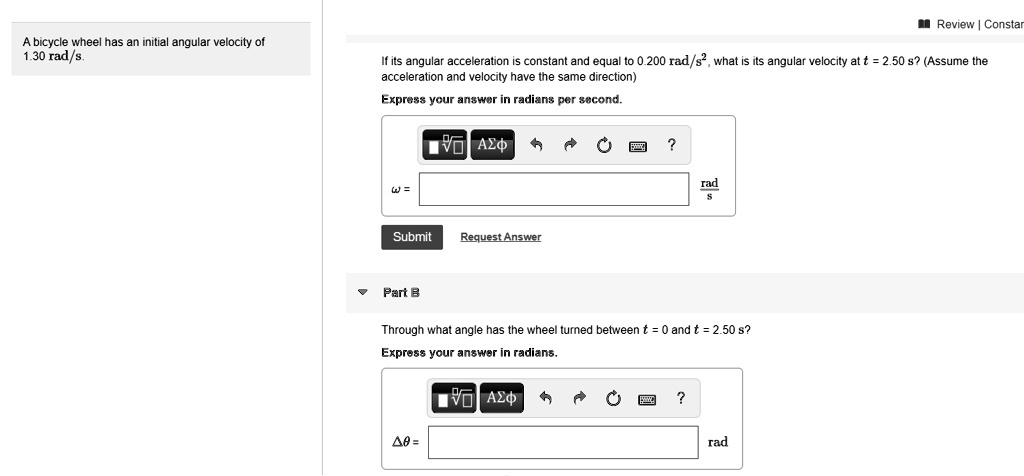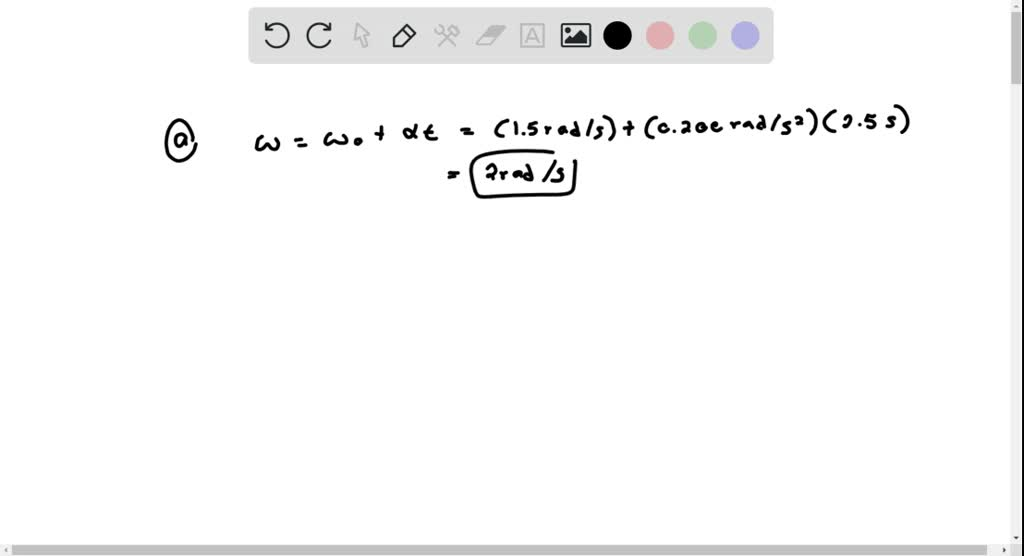5

# Review Constarbicycle wheel has an initial angular velocity of 30 radIf its angular acceleration constant and equal 0 200 rad/s? what is its angular velocity att = ...

## Question

###### Review Constarbicycle wheel has an initial angular velocity of 30 radIf its angular acceleration constant and equal 0 200 rad/s? what is its angular velocity att = 2.50 5? (Assume the acceleration and velocity have the same direction)Fxpress your answer in radiang per secondAEdradSubmitRequest AnswerPar BThrough what angle has the wheel turned between Expross Vour answvor radiansand2.50 $?Azq4e =rad Review Constar bicycle wheel has an initial angular velocity of 30 rad If its angular acceleration constant and equal 0 200 rad/s? what is its angular velocity att = 2.50 5? (Assume the acceleration and velocity have the same direction) Fxpress your answer in radiang per second AEd rad Submit Request Answer Par B Through what angle has the wheel turned between Expross Vour answvor radians and 2.50$? Azq 4e = rad